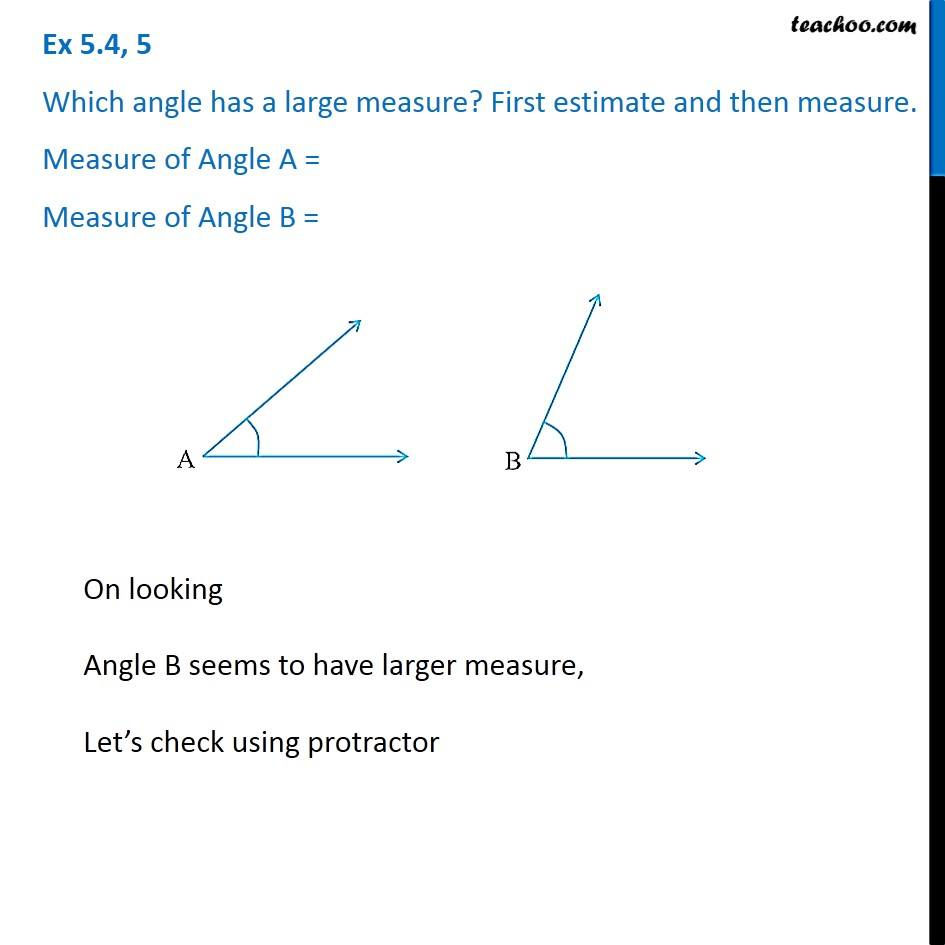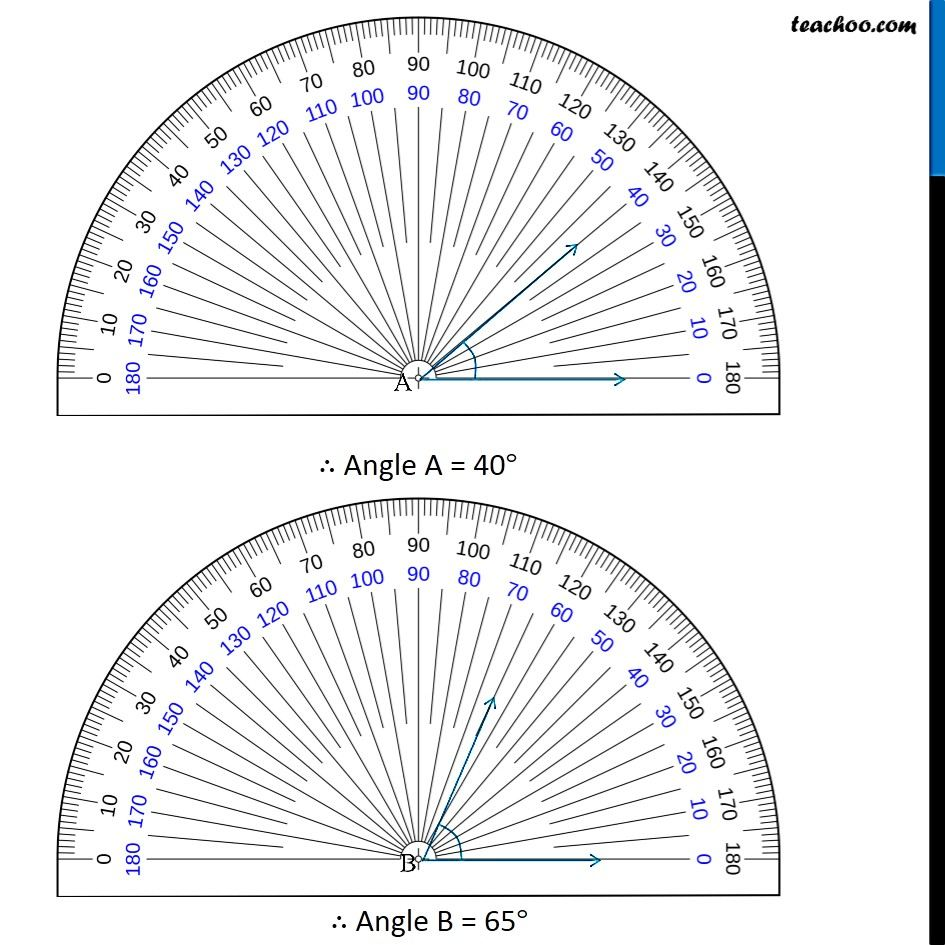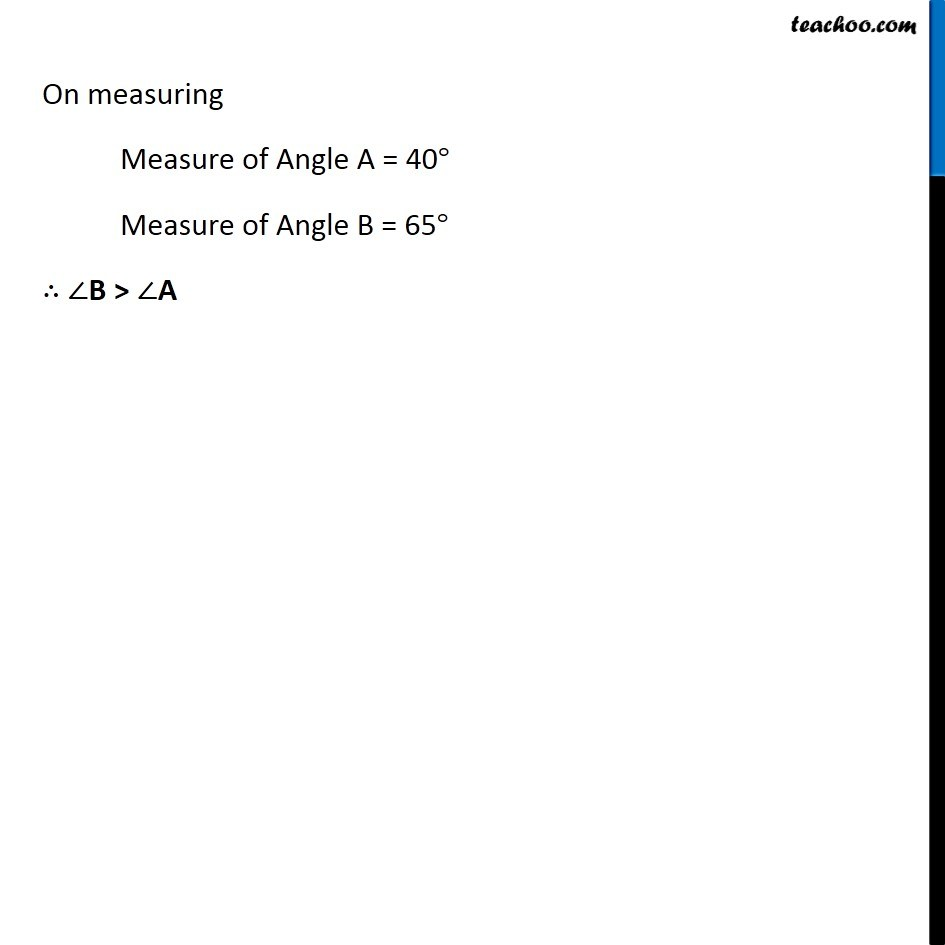Ex 5.4

Chapter 5 Class 6 Understanding Elementary Shapes
Serial order wiseLearn in your speed, with individual attention - Teachoo Maths 1-on-1 Class

### Transcript

Ex 5.4, 5 Which angle has a large measure? First estimate and then measure. Measure of Angle A = Measure of Angle B = On looking Angle B seems to have larger measure, Let’s check using protractor On measuring Measure of Angle A = 40° Measure of Angle B = 65° ∴ ∠B > ∠A